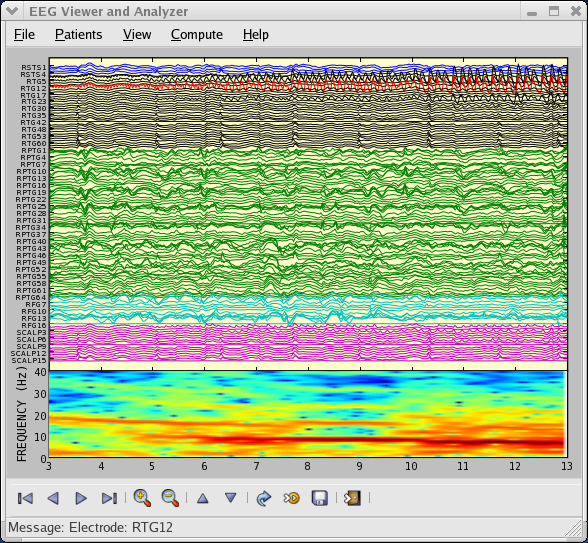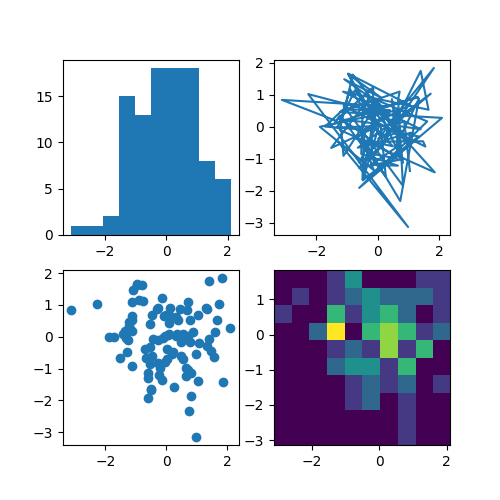Travis-CI:# Sample plots in Matplotlib¶

Here you’ll find a host of example plots with the code that generated them.

## Line Plot¶

Here’s how to create a line plot with text labels using `plot()`.

## Multiple subplots in one figure¶

Multiple axes (i.e. subplots) are created with the `subplot()` function:

## Images¶

Matplotlib can display images (assuming equally spaced horizontal dimensions) using the `imshow()` function.

## Contouring and pseudocolor¶

The `pcolormesh()` function can make a colored representation of a two-dimensional array, even if the horizontal dimensions are unevenly spaced. The `contour()` function is another way to represent the same data:

## Histograms¶

The `hist()` function automatically generates histograms and returns the bin counts or probabilities:

## Paths¶

You can add arbitrary paths in Matplotlib using the `matplotlib.path` module:

## Three-dimensional plotting¶

The mplot3d toolkit (see Getting started and mplot3d toolkit) has support for simple 3d graphs including surface, wireframe, scatter, and bar charts.

Thanks to John Porter, Jonathon Taylor, Reinier Heeres, and Ben Root for the `mplot3d` toolkit. This toolkit is included with all standard Matplotlib installs.

## Streamplot¶

The `streamplot()` function plots the streamlines of a vector field. In addition to simply plotting the streamlines, it allows you to map the colors and/or line widths of streamlines to a separate parameter, such as the speed or local intensity of the vector field.

This feature complements the `quiver()` function for plotting vector fields. Thanks to Tom Flannaghan and Tony Yu for adding the streamplot function.

## Ellipses¶

In support of the Phoenix mission to Mars (which used Matplotlib to display ground tracking of spacecraft), Michael Droettboom built on work by Charlie Moad to provide an extremely accurate 8-spline approximation to elliptical arcs (see `Arc`), which are insensitive to zoom level.

## Bar charts¶

Use the `bar()` function to make bar charts, which includes customizations such as error bars:

You can also create stacked bars (bar_stacked.py), or horizontal bar charts (barh.py).

## Pie charts¶

The `pie()` function allows you to create pie charts. Optional features include auto-labeling the percentage of area, exploding one or more wedges from the center of the pie, and a shadow effect. Take a close look at the attached code, which generates this figure in just a few lines of code.

## Tables¶

The `table()` function adds a text table to an axes.

## Scatter plots¶

The `scatter()` function makes a scatter plot with (optional) size and color arguments. This example plots changes in Google’s stock price, with marker sizes reflecting the trading volume and colors varying with time. Here, the alpha attribute is used to make semitransparent circle markers.

## GUI widgets¶

Matplotlib has basic GUI widgets that are independent of the graphical user interface you are using, allowing you to write cross GUI figures and widgets. See `matplotlib.widgets` and the widget examples.

## Filled curves¶

The `fill()` function lets you plot filled curves and polygons:

Thanks to Andrew Straw for adding this function.

## Date handling¶

You can plot timeseries data with major and minor ticks and custom tick formatters for both.

See `matplotlib.ticker` and `matplotlib.dates` for details and usage.

## Log plots¶

The `semilogx()`, `semilogy()` and `loglog()` functions simplify the creation of logarithmic plots.

Thanks to Andrew Straw, Darren Dale and Gregory Lielens for contributions log-scaling infrastructure.

## Polar plots¶

The `polar()` function generates polar plots.

## Legends¶

The `legend()` function automatically generates figure legends, with MATLAB-compatible legend-placement functions.

Thanks to Charles Twardy for input on the legend function.

## TeX-notation for text objects¶

Below is a sampling of the many TeX expressions now supported by Matplotlib’s internal mathtext engine. The mathtext module provides TeX style mathematical expressions using FreeType and the DejaVu, BaKoMa computer modern, or STIX fonts. See the `matplotlib.mathtext` module for additional details.

Matplotlib’s mathtext infrastructure is an independent implementation and does not require TeX or any external packages installed on your computer. See the tutorial at Writing mathematical expressions.

## Native TeX rendering¶

Although Matplotlib’s internal math rendering engine is quite powerful, sometimes you need TeX. Matplotlib supports external TeX rendering of strings with the usetex option.

## EEG GUI¶

You can embed Matplotlib into pygtk, wx, Tk, or Qt applications. Here is a screenshot of an EEG viewer called pbrain.The lower axes uses `specgram()` to plot the spectrogram of one of the EEG channels.

For examples of how to embed Matplotlib in different toolkits, see:

## XKCD-style sketch plots¶

Just for fun, Matplotlib supports plotting in the style of `xkcd`.

## Subplot example¶

Many plot types can be combined in one figure to create powerful and flexible representations of data.

```import matplotlib.pyplot as plt
import numpy as np

np.random.seed(19680801)
data = np.random.randn(2, 100)

fig, axs = plt.subplots(2, 2, figsize=(5, 5))
axs[0, 0].hist(data)
axs[1, 0].scatter(data, data)
axs[0, 1].plot(data, data)
axs[1, 1].hist2d(data, data)

plt.show()
```Total running time of the script: ( 0 minutes 0.082 seconds)

Gallery generated by Sphinx-Gallery